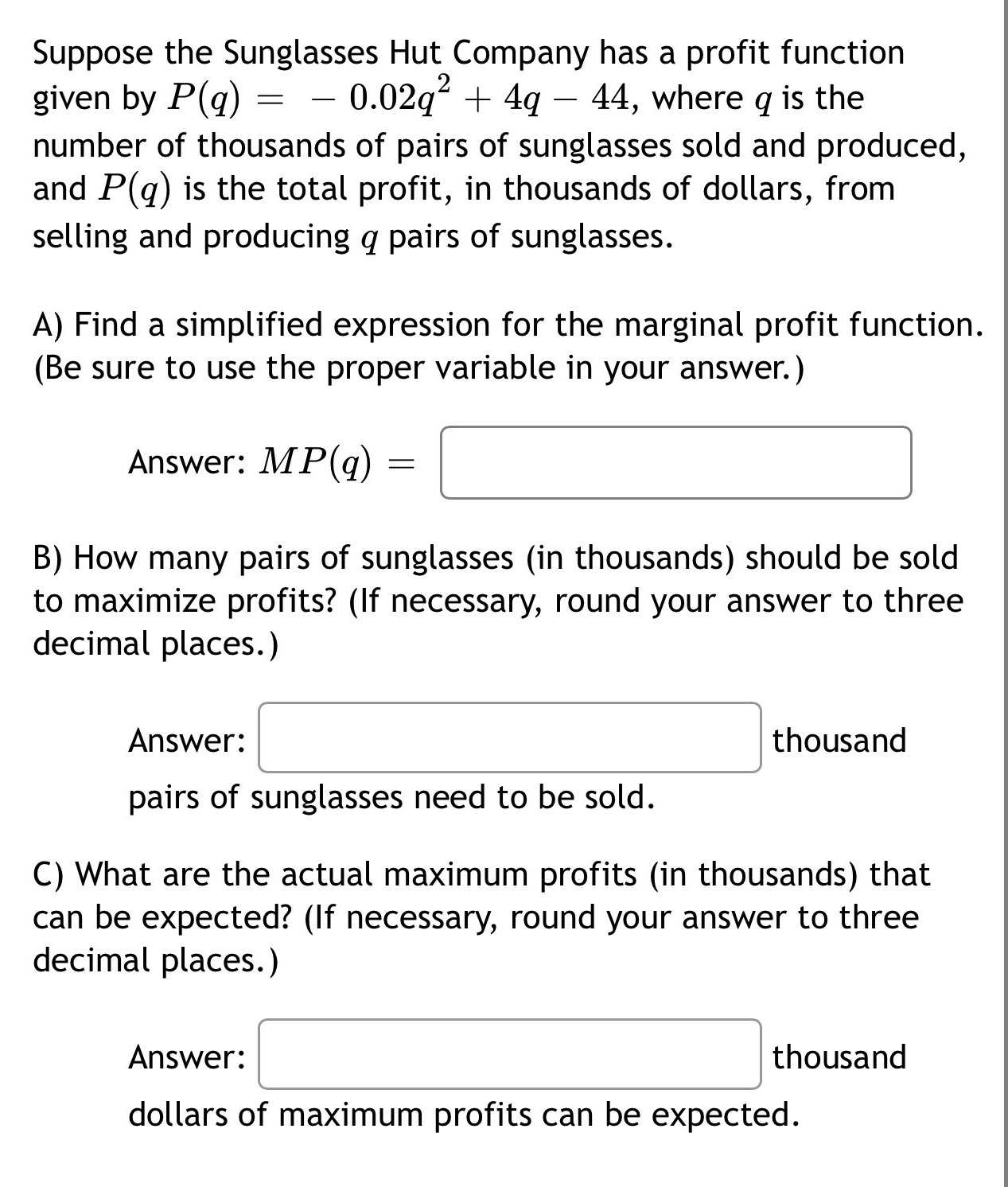### Still have math questions?

Algebra
QuestionSuppose the Sunglasses Hut Company has a profit function given by $$P ( q ) = - 0.02 q ^ { 2 } + 4 q - 44$$ , where $$q$$ is the number of thousands of pairs of sunglasses sold and produced, and $$P ( q )$$ is the total profit, in thousands of dollars, from selling and producing $$q$$ pairs of sunglasses.

A) Find a simplified expression for the marginal profit function. (Be sure to use the proper variable in your answer.)

Answer: $$M P ( q ) = \square$$

B) How many pairs of sunglasses (in thousands) should be sold to maximize profits? (If necessary, round your answer to three decimal places.)

Answer:  $$\square$$ thousand pairs of sunglasses need to be sold.

C) What are the actual maximum profits (in thousands) that can be expected? (If necessary, round your answer to three decimal places.)

Answer: $$\square$$ thousand dollars of maximum profits can be expected.

A. $$MP_{(q)}= -0.04q+ 4$$
B. $$q = 100,000\ sunglasses$$
C. $$Maximum\ Profit = 156,000$$